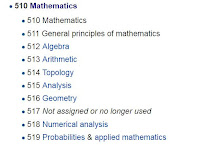## Sunday, September 25, 2016

### Classifying Mathematics

As an idle curiosity for some time, I've been trying to get my head around all the areas of the field of mathematics, and how to categorize them.

1. So first there's the way they are approached in education:
• arithmetic (numbers and their relations)
• algebra (I took in the 8th and 10th grades)
• geometry ( I took in the 9th grade)
• analytical geometry and trigonometry (I took in the 11th grade)
• calculus 1 and 2 (I took in the 12th grade)
• calculus 3 (series, summations) and differential equations (I took first year of college)
• My step-daughters have taken math courses I haven't: finite math and statistics.
• I remember hearing about number theory in high school, which speaks patterns and tricks among numbers (prime numbers, how to tell if a number is divisible by 3 or 6, etc...)
2. Then I got called into ministry, but the Duke University math department seems to lay out what the rest of an undergraduate math degree would look like:
• Linear Algebra (matrix algebra, vector spaces)
• Abstract Algebra (group theory, ring theory)
• Complex Analysis (doing calculus with imaginary numbers)
• Topology, Differential geometry (this is geometry stuff on steroids)
• Set theory
3. I also looked at how the Dewey Decimal System and the Library of Congress classify math.Dewey Decimal SystemLibrary of Congress

4. So here's my attempt to organize math in my own way.

I. Quantities and Their Relationships
• arithmetic
• number theory
• set theory and logic
• probability, statistics, combinatorics (finite)
• series and summations (infinites)
• analysis (change: differential and integral calculus, complex analysis)
II. Spaces
• geometry
• analytical geometry
• trigonometry
• differential geometry
• topology
III. Tools
• algebra
• linear algebra (including matrix algebra)
• abstract algebra (group theory, ring theory)
• differential equations
• numerical analysis
OK, math people, critique?